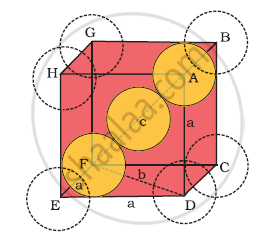Department of Pre-University Education, KarnatakaPUC Karnataka Science Class 12

# Calculate the Efficiency of Packing in Case of a Metal Crystal For Body-centred Cubic - Chemistry

Calculate the efficiency of packing in case of a metal crystal for body-centred cubic

#### Solution

Body-centred cubic:It can be observed from the above figure that the atom at the centre is in contact with the other two atoms diagonally arranged.

From ΔFED, we have:

b2 = a2 + a2

⇒ b2 =  2a2

⇒ b = sqrt2a

Again, from ΔAFD, we have:

c2 = a2 + b2

⇒ c2 = a2 + 2a2     (Since b2 = 2a2)

⇒ c2 = 3a2

⇒ c = sqrt3a

Let the radius of the atom be r.

Length of the body diagonal, c = 4π

⇒sqrt3a = 4r

⇒a =(4r)/sqrt3

or r = (sqrt3a)/4

Volume of the cube a^3 = ((4r)/sqrt3)^3

A body-centred cubic lattice contains 2 atoms.

So, volume of the occupied cubic lattice 2pi4/3 r^3

=8/3pir^3

:.Packing efficiency = ("Voulume occupied by two spheres in the unit cell")/"Total volume of unit"xx100%

= (8/3pir^3)/(4/(sqrt3)r)^3xx100%

=(8/3pir^3)/(64/(3sqrt3)r^3) xx 100%

=68%

Concept: Packing Efficiency - Efficiency of Packing in Body-centred Cubic Structures
Is there an error in this question or solution?
Chapter 1: The Solid State - Exercises [Page 31]

#### APPEARS IN

NCERT Class 12 Chemistry
Chapter 1 The Solid State
Exercises | Q 10.2 | Page 31
Share